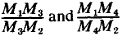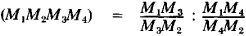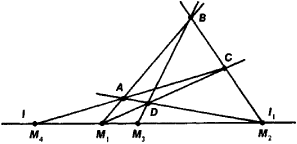# Harmonic Distribution

The following article is from The Great Soviet Encyclopedia (1979). It might be outdated or ideologically biased.

## Harmonic Distribution

an arrangement of four points, M1, M2, M3, and M4 on a straight line ll1 (see Figure 1) such that the point M3 lies within the interval M1M2, the point M4 is outside of this interval, and the ratiosare equal. Usually the ratio of two intervals is considered to be positive if their directions on the straight line are the same, and negative when the directions are different. Accordingly, the harmonic distribution of the points M1, M2, M3, and M4 can be described by the so-called cross-ratio of thesewhich is equal to −1 in the case of harmonic distribution. When a harmonic set of points is projected onto any straight line, it projects into a harmonic set of points. Consequently, harmonic distribution is one of the fundamental concepts of projective geometry. The following geometric configuration is associated with the harmonic distribution of the points M1, M2, M3, and M4. Let the points M1 and M2 be the points of intersection of the conjugate opposite sides of the quadrilateral ABCD. Then points M3 and M4 will be the points of intersection for the diagonals of this quadrilateral with the straight line M1, M2. (See Figure 1.)The concept of harmonic distribution carries over into other geometric objects: four rays projecting a harmonic set of points from one point form a harmonic family of rays. A harmonic family of planes is defined in similar fashion.

E. G. POZNIAK